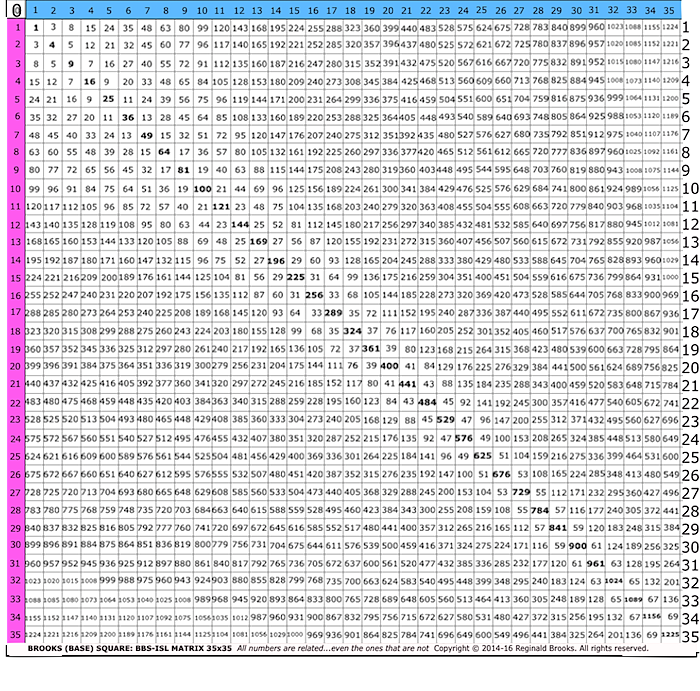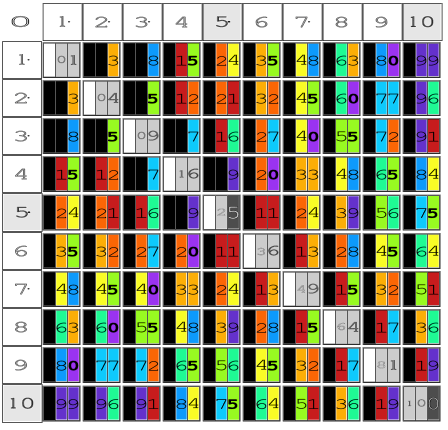# BBS-ISL Matrix Fundamentals:

### 10 Basic, fundamental rules of the symmetrical BBS-ISL Matrix

• Basic BBS-ISL Rule 1: All numbers (#s) related by the 1–4–9-…PD sequence

• Basic BBS-ISL Rule 2: Every # in the PD sequence is the square of an Axial #.

• Basic BBS-ISL Rule 3: The Odd-Number Summation sequence forms the PD sequence.

• Basic BBS-ISL Rule 4: Every EVEN Inner Grid (IG) # is divisible by 4 & all are present.

• Basic BBS-ISL Rule 5: Every IG# is:
• A: The difference (∆) between its two PD-sequence #s. (Note: A=B=C=D=E, and F.)

• Ex: \[PD 25 - PD9 = 16\]
• B: The sum (∑) of the ∆s of each of its PD#s between its two PD-sequence #s (as above).

• Ex:\[ (PD 25 - PD16) + (PD16 - PD9) = 16\]
• C: The ∆ between the squares of the two Axial #s forming that IG# (as above).

• Ex:\[5^2 - 3^2 = 16\]
• D: The product of the Addition & Subtraction of the two Axial #s forming that IG# (as above).

• Ex:\[(5 + 3) x (5 - 3) = 16\]
• E: The product of the Diagonal Axis # — STEPS from the PD — times the ∑ of Row + Column Axis #s.

• Ex:\[2 x (5 + 3) = 16\]
• F: Also, the product of its 2 Axial #s intersected by that IG#’s 90° diagonals.

• Ex:\[2 x 8 = 16\]
• Basic BBS-ISL Rule 6: Every *ODD IG# is NOT PRIME & all are present.
• Corollary: NO PRIME #s are present on the *IG.
• Corollary: NO EVEN, NOT divisible by 4, #s are present on the IG.
*Excepting the 3—5—7—… ODD #s of the 1st Parallel Diagonal
• Basic BBS-ISL Rule 7: The ODD-Number sequence, and the 1–4–9-…PD sequence, forms the sequential ∆ between ALL IG#s.

• Basic BBS-ISL Rule 8: The ∆ between #s within the Parallel Diagonals is a constant 2 x its Axial #.

• Basic BBS-ISL Rule 9: The ∆ between #s in the Perpendicular Diagonals follow:
• A: From EVEN PD#s, √PD x 4 starts the sequence & follows x1—x2—x3—x4….
• B: From ODD PD#s, √PD x 4 starts the sequence & follows x1—x2—x3—x4….
• C: From ODD Perpendicular Diagonals between the EVEN-ODD diagonals (above), the sequence starts with the same value as the Axis number ending the diagonal, the sequence following x1—x3—x5—x7..
• Basic BBS-ISL Rule 10: Every #, especially the #s in the ONEs Column, informs both smaller and larger Sub-set symmetries (much larger grids required to demonstrate).

# BBS-ISL Matrix Inner Grid Golden Rules (IGGR)

### 5 Basic, fundamental rules of the symmetrical BBS-ISL Matrix Inner Grid

• IGGR 1: The IG is formed of two equal & symmetrical 90°-right, isosceles triangles that are bilaterally symmetrical about the PD — and, infinitely expandable.

• IGGR 2: The 90°-right-triangle — inherent to ALL squares and rectangles by definition — both forms the alternating EVEN-ODD square grid cells within the Matrix, and, is responsible for all major patterns and sequences, thereupon.

• IGGR 3: Subtraction-Addition: Every IG# is simply the ∆ between its two PD#s (subtraction), and, the sum (∑) of any IG# + its PD# above = the PD# on the end of that Row (or, Column).

• IGGR 4: Multiplication-Division: Every IG# is simply the product of the two AXIAL #s intersected by the two diagonals — of that said IG# — pointing back to the Axis at a 90° angle (multiplication), and, the dividend of the Axial divisor and quotient (division).

• IGGR 5: The actual # of grid-cell steps — i.e., the actual # of STEPS from a given IG# to another by a strictly horizontal, vertical, or 45° diagonal path — forms a simple, yet often fundamental descriptor to the pattern-sequence templates that inform the more advanced patterns, e.i., Exponentials and especially the Pythagorean Triples (PTs). STEPS are particularly important in the geometric visualizations within the BBS-ISL Matrix (as alluded to in IGGR 2, above).

# Pythagorean Triples and BBS-ISL Fundamentals (TPISC: The Pythagorean-Inverse Square Connection)

### 3 Basic, fundamental rules of the symmetrical BBS-ISL Matrix Inner Grid that encompass the PTs.

• TPISC-BBS-ISL Rule 1: Every IG EVEN Squared # is part of a Paired-Factor Set (PFS) that:
• A: Has reciprical PFS members on the PD vertically above.
• B: Both PFS members reside on the SAME Row.
• C: They represent the a2 and b2 values of a PT, whose c2 value is on the PD intersection
• TPISC-BBS-ISL Rule 2: Every PT is found on the BBS-ISL Matrix and can be located by this intersection of EVERY PD (9>) and a Row with PFSs.

• TPISC-BBS-ISL Rule 3: Every PT — including its sides, perimeter, area and proof — can also be found and fully profiled (and, predicted) as r-set, s-,t-set members of the Dickson Method (DM), Expanded Dickson Method (EDM), and the Fully Expanded Dickson Method (FEDM), shown herein.#### ImagesKEYWORDS TAGS: TPISC, The Pythagorean - Inverse Square Connections, Pythagorean Triangles, Pythagorean Triples, primitive Pythagorean Triples, non-primitive Pythagorean Triples, Pythagorean Theorem, Pythagorus Theorem, The Dickson Method, BBS-ISL Matrix, Expanded Dickson Method, r-sets, s-set, t-sets, Pair-sets, geometric proofs, MathspeedST, leapfrogging LightspeedST FASTER than the speed of light, Brooks (Base) Square- Inverse Square Law (ISL), BBS-ISL Matrix grid, The Architecture Of SpaceTime (TAOST), The Conspicuous Absence Of Primes (TCAOP), A Fresh Piece Of Pi(e), AFPOP, Numbers of Inevitability, LightspeedST, Teachers, Educators and Students (TES), number theory, ubiquitous information, FASTER than the speed of light, primes, prime numbers, fractals, mathematics, Universe, cosmos, patterns in number.

Art Theory 101 / White Papers Index    |   New Media net.art index    |   home